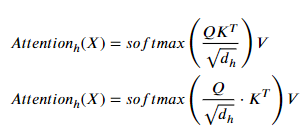# Why denominator in multi-head attention in PyTorch's implementation different from most proposed structure?

As the title describes. I just compared the code of transformers from hugging face’s Bert and torch.nn.Transformer, directly from the code I found in my site-packages. Specifically, I’m looking for the part of dividing attention score before softmax. Most paper on transformer mentioned this:In hugging face’s Bert, I believe it is this line of code, which implement this quite right.

I believe the closest thing in PyTorch is this line, which is actually dividing query, not the result of dot product.

I’d like to know if they’re really equivalent? Wouldn’t that change the amount of backpropagation into Wq and Qk?
I know training transformer from scratch is hard. I’m having trouble training torch.nn.Transformer from scratch in a recent project. But could this be a reason why the loss won’t go down?

Since 𝑑_ℎ represents a scalar, it should not matter if you do it before or after the matrix multiplication.Of course due to resolution you will get slightly different results, but I do not think that this should be enough reason for your loss to not go down. Here is a small example to show that there are differences, but they are very small.

``````import torch
import math

q = torch.rand(1, 5, 30)
k = torch.rand(1, 5, 30)
v = torch.rand(1, 5, 30)

B, Nt, E = q.shape
q_ = q / math.sqrt(E)
attn1 = torch.bmm(q_, k.transpose(-2, -1))
attn2 = torch.bmm(q, k.transpose(-2, -1)) / math.sqrt(E)

print(f"Attn1: {attn1}")
print(f"Attn2: {attn2}")
print(f"Compa: {attn1==attn2}")
``````
``````# Output:
Attn1: tensor([[[1.4470, 1.2312, 1.4192, 1.0483, 1.0938],
[1.5067, 1.2858, 1.3447, 1.3185, 1.2335],
[1.3196, 1.1793, 1.3776, 1.1048, 1.0500],
[1.2411, 1.1090, 1.3351, 0.9647, 0.9519],
[1.6056, 1.5329, 1.6754, 1.3193, 1.4312]]])
Attn2: tensor([[[1.4470, 1.2312, 1.4192, 1.0483, 1.0938],
[1.5067, 1.2858, 1.3447, 1.3185, 1.2335],
[1.3196, 1.1793, 1.3776, 1.1048, 1.0500],
[1.2411, 1.1090, 1.3351, 0.9647, 0.9519],
[1.6056, 1.5329, 1.6754, 1.3193, 1.4312]]])
Compa: tensor([[[ True, False, False, False,  True],
[False,  True,  True,  True, False],
[ True, False, False, False,  True],
[False,  True,  True, False, False],
[False, False, False,  True, False]]])
``````

Transformers do need a lot of training data and a lot of time to train. Would it be possible for you to take a pretrained model and fine tune it to your specific task?

1 Like